Computer Science

# A Tutorial on Decomposition

This tutorial will show you how to:

• decompose a problem into a set of functions,
• work on one function at a time, and
• use variables

## The problem

We are going to work on the lines problem from Lab 8. In this problem, Bit needs to connect each square of the same color on each line. The starting world looks like this: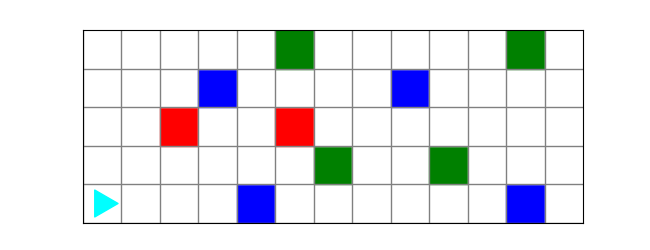and the finished world should look like this: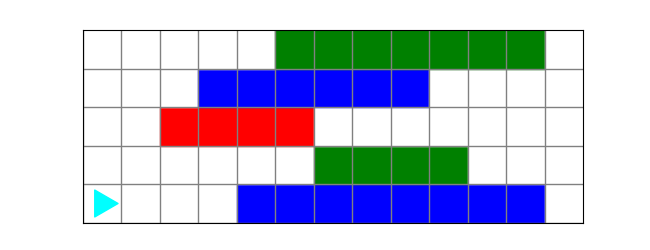## Start small

Start by thinking about how to do just one line. How would you get Bit to connect the colored squares on the bottom row?

from byubit import Bit

@Bit.run('lines')
def run(bit):
# connect the colored squares on the first row
find_a_color_and_make_a_line(bit)

This function doesn’t exist yet, but we can imagine it does exactly what we want, and we just need to call it. Now decompose that function into pieces. Draw a picture: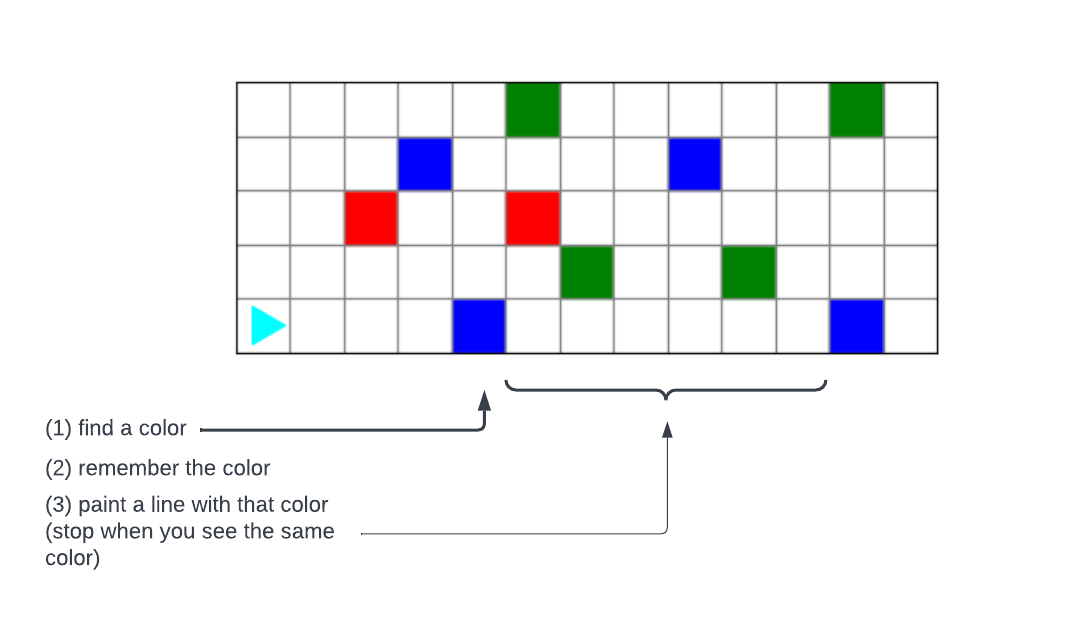Use comments to explain what you need.

def find_a_color_and_make_a_line(bit):
# find a color
# remember the color
# paint a line with that color (stop when you see the same color)

And then write that code:

def find_a_color_and_make_a_line(bit):
# find a color
while bit.is_empty():
bit.move()
# remember the color
color = bit.get_color()
# paint a line with that color (stop when you see the same color)
bit.move()
while bit.get_color() != color:
bit.paint(color)
bit.move()

(1) We use bit.is_empty() to check whether the current square has no color. We want to keep moving until we find a square that has a color. We don’t know in advance what color that will be, because we could be working on any line.

(2) We use color = bit.get_color() to get the color of the square bit is currently on, and then store that color in a variable called color. You can call this variable anything you want — such as puppy — but it makes sense to use a name that indicates what the variable references.

(3) Once we have the color variable, we can keep painting that color until we reach a square with the same color. You have to be careful to paint and then move, so you can check if you have reached the end before you keep painting and moving.

Try running this code. If it doesn’t work, figure out the problem and fix it.

Note: when we wrote this code in class, it had bugs! Sometimes a class member noticed them before we ran the code — that’s the power of coding in a team. Sometimes everyone missed it. That’s OK. Since you are working on one function at a time, debugging this function is a fairly simple problem. It’s a lot easier than writing all the code for the whole problem before trying any of it.

Once you have the code working, you can be confident that the first piece of the problem is solved. Don’t worry about the fact that the rest of the problem isn’t working. We haven’t even tried that part yet!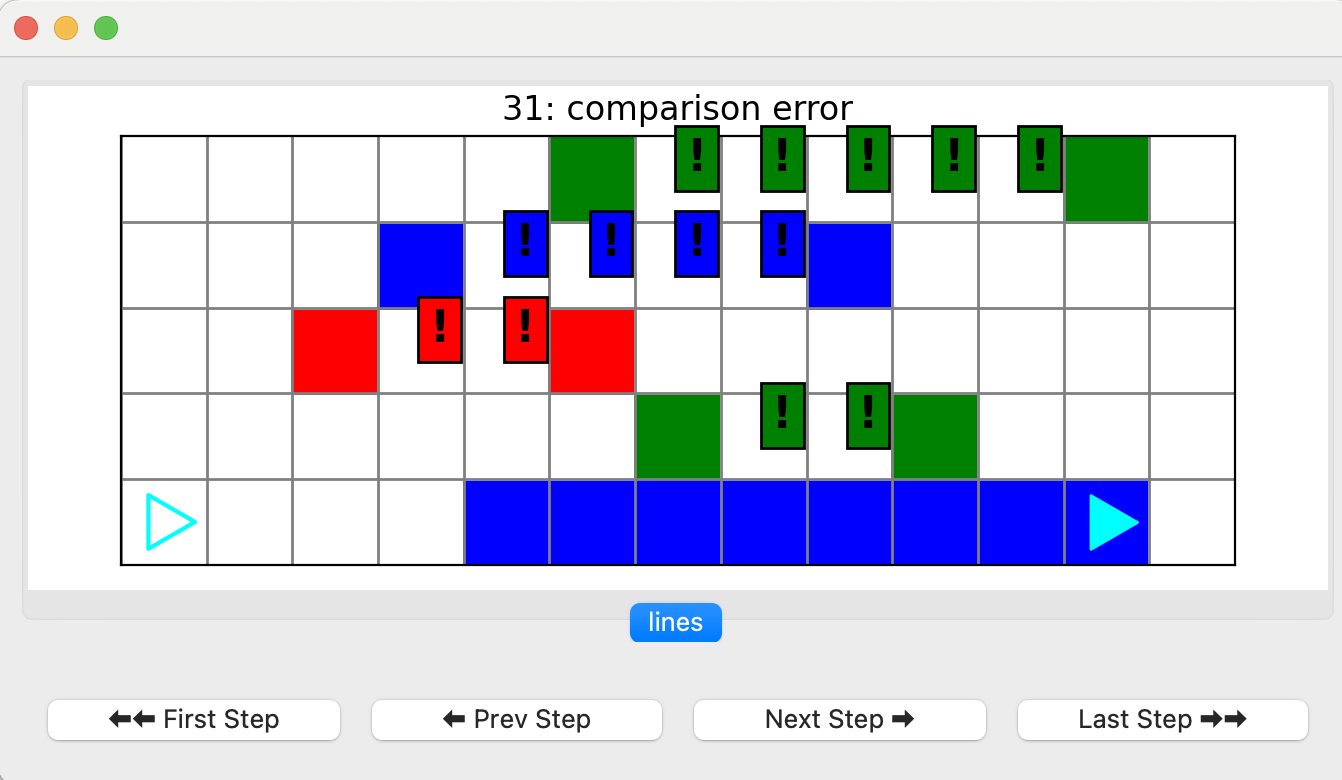## Solve the next piece

Now that you have this working, the next piece is to turn around and go back to the beginning of the line. This will allow Bit to move up a line in case there is another line to work on.

@Bit.run('lines')
def run(bit):
# connect the colored squares on the first row
find_a_color_and_make_a_line(bit)
# turn around and go back
turn_around_and_go_back(bit)

We have written functions like this before! It is fairly simple to turn Bit around and go back the other direction:

def turn_around_and_go_back(bit):
bit.right()
bit.right()
while bit.front_clear():
bit.move()
bit.right()

Notice that we turn right so we are pointing up after we go back. This is because we know we want to check if there is a row above Bit, and we can do this by checking whether the front is clear.

Check this code before moving on!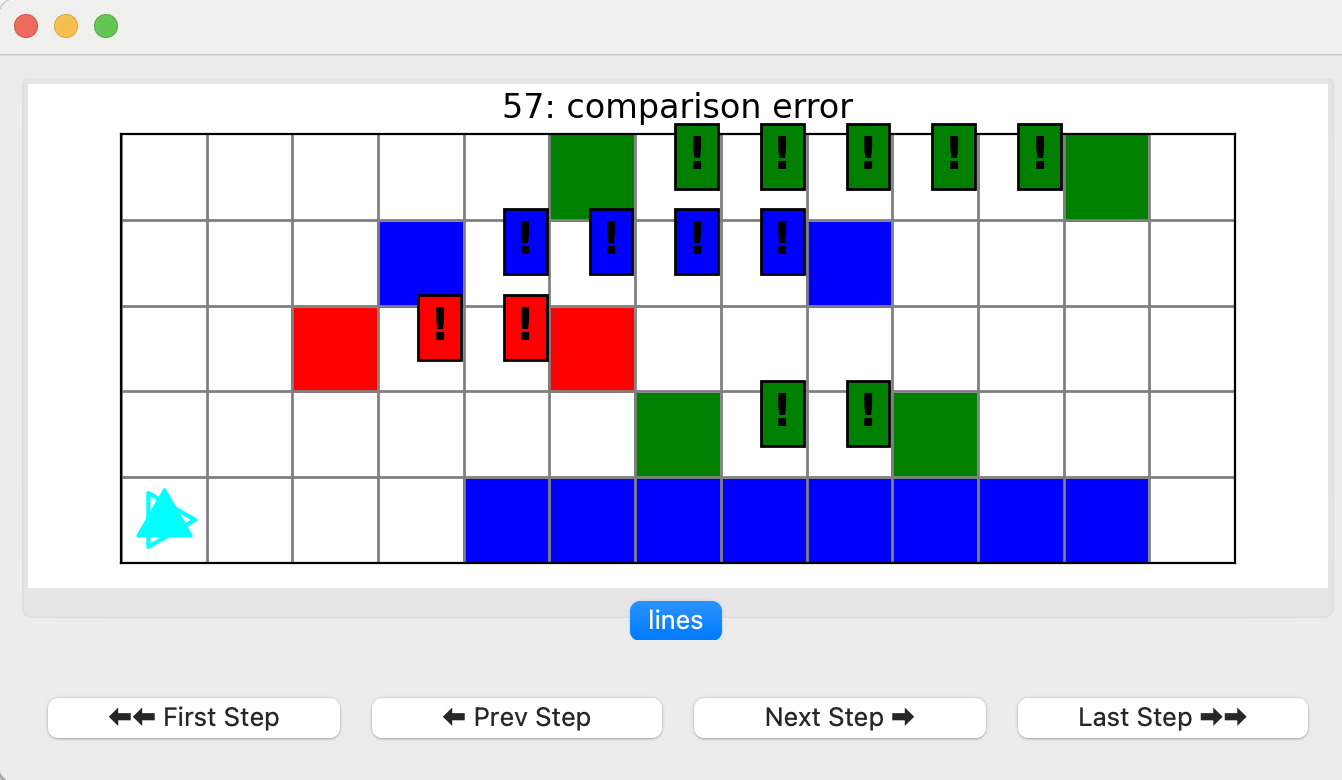## Looping

We have the important pieces of this puzzle done. We can do a single line. We can use these pieces to build a loop to do all the lines:

@Bit.run('lines')
def run(bit):
# Implement
# connect the colored squares on the first row
find_a_color_and_make_a_line(bit)
# turn around and go back
turn_around_and_go_back(bit)
# while if there is room for another row...
while bit.front_clear():
# ... move into that row
move_into_row(bit)
# then repeat what we did on the first row
find_a_color_and_make_a_line(bit)
turn_around_and_go_back(bit)

In this code, we are calling another function that doesn’t exist yet — move_into_row(). Let’s write that function:

def move_into_row(bit):
bit.move()
bit.right()

It’s pretty simple! Don’t be afraid to write small functions like this.

If we run the code we have so far, we are almost done!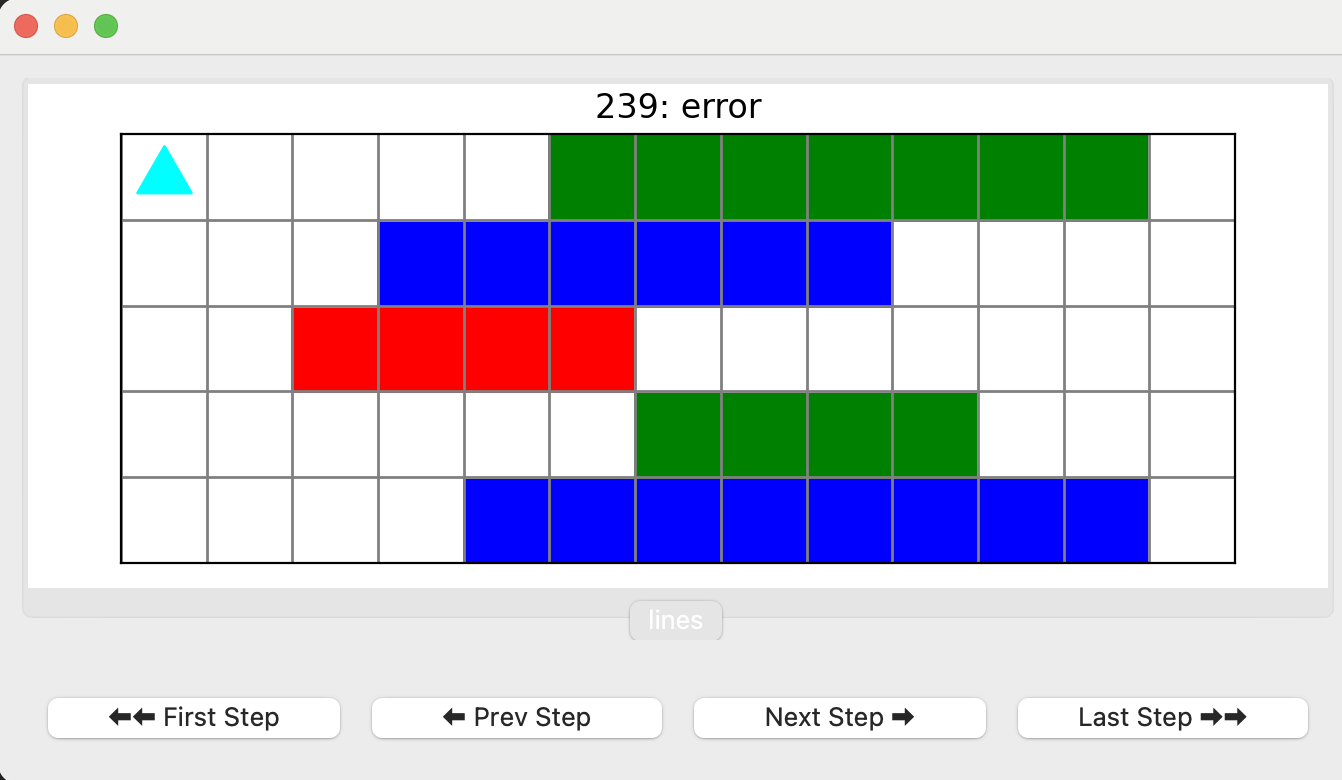## The last piece

Now we just need to go back home

@Bit.run('lines')
def run(bit):
# Implement
# connect the colored squares on the first row
find_a_color_and_make_a_line(bit)
# turn around and go back
turn_around_and_go_back(bit)
# while if there is room for another row...
while bit.front_clear():
# ... move into that row
move_into_row(bit)
# then repeat what we did on the first row
find_a_color_and_make_a_line(bit)
turn_around_and_go_back(bit)

go_back_to_the_beginning(bit)

We need to write a function that can go back to the beginning.

def go_back_to_the_beginning(bit):
turn_around_and_go_back(bit)
bit.right()
bit.right()

It turns out we can use the turn_around_and_go_back() function we wrote previously, and then just turn to make Bit face the right direction.

Everything works!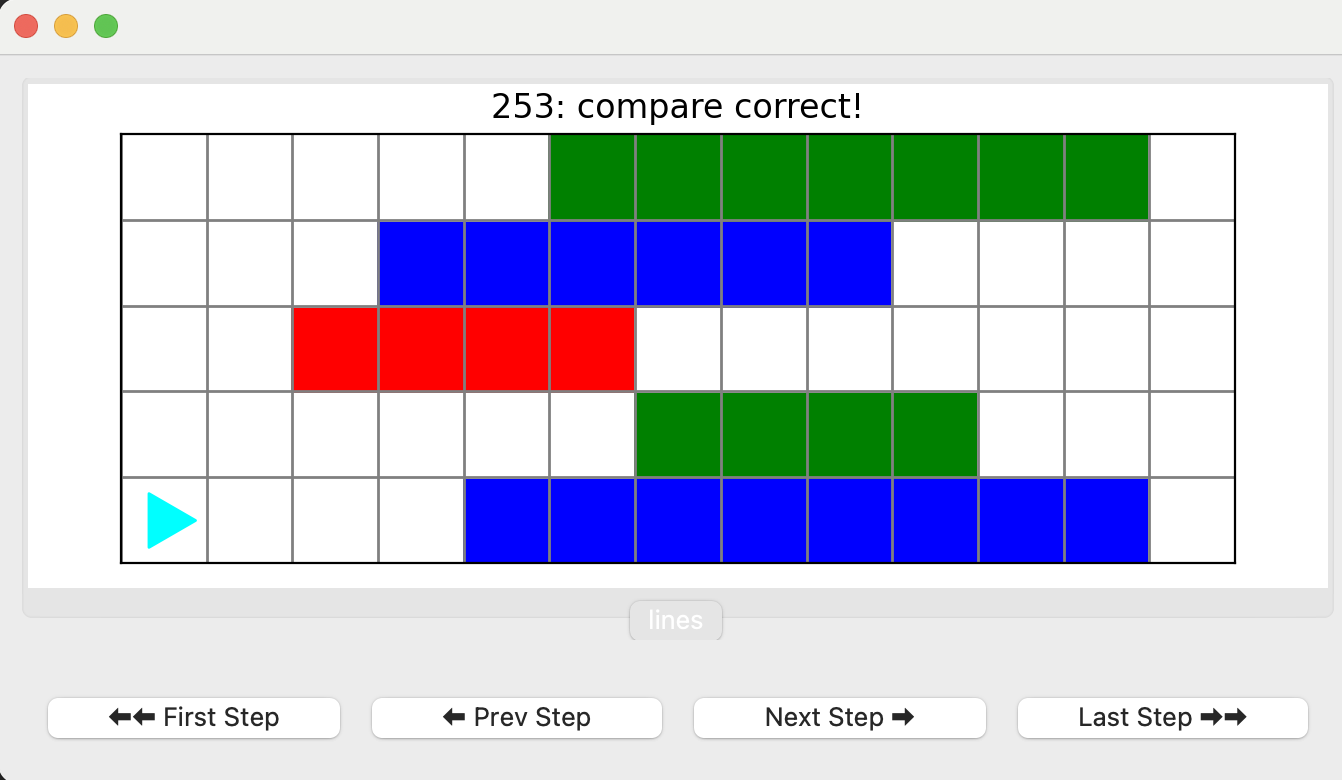## Refactoring

If you look back at the find_a_color_and_make_a_line() function, it is a little long:

def find_a_color_and_make_a_line(bit):
# find a color
while bit.is_empty():
bit.move()
# remember the color
color = bit.get_color()
# paint a line with that color (stop when you see the same color)
bit.move()
while bit.get_color() != color:
bit.paint(color)
bit.move()

We could refactor this code — rewrite it without changing what it does — by creating a make_a_line() function that does that second while loop:

def make_a_line(bit, color):
bit.move()
while bit.get_color() != color:
bit.paint(color)
bit.move()

def find_a_color_and_make_a_line(bit):
# find a color
while bit.is_empty():
bit.move()
# remember the color
color = bit.get_color()
# paint a line with that color (stop when you see the same color)
make_a_line(bit, color)

Notice how make_a_line() takes two parameters — a bit world and a color.

We could also write a find_a_color() function that handles the first while loop:

def find_a_color(bit):
while bit.is_empty():
bit.move()

def make_a_line(bit, color):
bit.move()
while bit.get_color() != color:
bit.paint(color)
bit.move()

def find_a_color_and_make_a_line(bit):
# find a color
find_a_color(bit)
# remember the color
color = bit.get_color()
# paint a line with that color (stop when you see the same color)
make_a_line(bit, color)

These kinds of changes make the code a little easier to understand. In general, smaller functions are easier to write and easier to maintain.